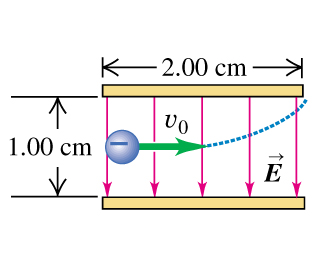# Problem: An electron is projected with an initial speed v0 = 1.10 × 106 m/s into the uniform field between the parallel plates in the figure. Assume that the field between the plates is uniform and directed vertically downward, and that the field outside the plates is zero. The electron enters the field at a point midway between the plates.The electron just misses the upper plate as it emerges from the field.(a) Suppose that in the figure, the electron is replaced by a proton with the same initial speed. Would the proton hit one of the plates?(b) What would be the direction of proton's displacement? Upward or downward?

###### FREE Expert Solution

(a)

Electric force :

$\overline{){\mathbf{F}}{\mathbf{=}}{\mathbf{qE}}}$

Newton's second law:

$\overline{){\mathbf{\Sigma }}{\mathbf{F}}{\mathbf{=}}{\mathbf{m}}{\mathbf{a}}}$

The charge on a proton and an electron is the same.

Thus, the magnitude of the electric force experienced by a proton and an electron is the same.

|Felectron| = |Fproton| = |eE|

Acceleration:

a = F/m###### Problem Details

An electron is projected with an initial speed v0 = 1.10 × 106 m/s into the uniform field between the parallel plates in the figure. Assume that the field between the plates is uniform and directed vertically downward, and that the field outside the plates is zero. The electron enters the field at a point midway between the plates.The electron just misses the upper plate as it emerges from the field.

(a) Suppose that in the figure, the electron is replaced by a proton with the same initial speed. Would the proton hit one of the plates?

(b) What would be the direction of proton's displacement? Upward or downward?